# Introduction

Hello, my name is Anthony Hosemann and I am an IT software development student at SAIT. This is my second blog on machine learning. In this blog post I will be demonstrating a supervised learning ML application that I created in java using a decision tree classifier.

# Review

In my last blog post I went over some of the basics of machine learning and supervised learning but In case you missed it or forgot some stuff I am going to do a quick review of some of the key concepts of supervised learning.

# Classifier

First let’s talk about the classifier. The classifier is the part of the application that makes decisions and chooses what the correct output should be for any given input data. In supervised learning the classifier must be trained by training data for it to be accurate. The classifier uses the training data to learn and create rules that will make it more accurate. There are lots of different kinds of machine learning classifiers but the one that I chose to use for the application in this blog post is the decision tree classifier.

# Training Data

Now before I get into the code of the application, I will show you the training data that I will be using in my demonstration: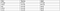Training data table

If you read my last blog post on machine learning you may recognize this data. This is the same table of fruit data that I used in my last blog post. This table contains a list of features and labels of various fruits which will be used to help the classifier learn what colors and textures correspond to what fruits. Now let’s look at what the training data looks like in code: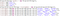Training data code

In this code I have created one row of data that I called headers which stores the headers for the table of data that is going to be used in the classifier. I also created a table of data by creating an ArrayList that holds rows of data (ArrayList<String>). After instantiating the 2D ArrayList called trainingData I then added the data row by row. All of the data I added to the 2D ArrayList is the same as in the training data table provided above.

# Coding the Classifier

So now what? We have some training data but no classifier to train. So, lets begin coding the classifier. This section is going to have a lot of code segments but if you would rather look at the entire application, I have uploaded it to github so you can see the code there as well. The classifier will need to be able to do a few things for it to be functional. These things would be:

- Getting unique values in each feature column. This is important because the training data in most cases is MUCH larger than just 5 records and there will be repeating data in some columns and we don’t want to have to loop through all of them whenever we create a question.

- Counting how many different unique labels are in a given set of data. We need this because it allows us to figure out if a question asked by the classifier helps reduce the gini impurity of the resulting data.

- We also need a way to calculate the gini impurity and information gain of any given set of data.

- Then we can use gini impurity and information gain to find the question that splits the data the best.

- Finally, we need a way to partition the data once we have found the question that splits the data the best.

First lets start with getting the unique features in a column. This can be done by Iterating through the training data and adding any unique values found into a new ArrayList<String>. Here is the code for this method: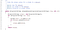uniqueValues method

The method used to count how many labels are in a given set of data is very similar to the uniqueValues method except now you will need to create an ArrayList<Integer> that will keep track of how many occurrences of each label there were. You will also not need to give the column number as a parameter as the label column is always the last column. This is the code for the countClass method: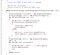countClass method

We also need methods to calculate gini impurity and information gain. This is the code used to calculate gini impurity: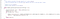gini method

And this is the code used to calculate information gain: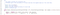infoGain method

Now we need to use the gini impurity and information gain to find the question that splits the data the best. Here is the code for the findBestSplit method: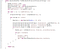findBestSplit method

Finally, we have one of the most important methods in the classifier which is the partition method that splits the data based on a question. Here is the code for the partition method: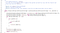partition method

# Building the Tree

Now that we have a table of data along with the beginnings of a classifier, we can now begin creating the methods for building the decision tree. To do this we will need Nodes that hold questions and references to the resulting true and false branches, I call the decision nodes. We will also need leaf Nodes that hold the predictions at the end of a branch. Finally, we need a recursive method that will build the tree with decision nodes and leaf nodes. This buildTree method will be the method that trains our classifier to identify certain types of objects in a dataset so this is where you would pass in your table of training data. Here is the code for the buildTree method: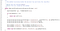buildTree method

Now that we have a tree built, we can print it using another recursive method called printTree. The way that I built this method prints out the tree like this: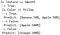printTree output

This tree was built using the fruit training data that I provided earlier in this blog. As the method goes farther into the true/false branches the printTree method will add extra spaces to the beginning of each String to help clarify where in the tree you are looking at.

# Using the classifier

Now we have almost finished creating the classifier. The last thing the classifier needs to be able to do is take in an unknown row of data and output a prediction on what object the data represents. I called this method classify and it takes in an unknown row of data and the decision tree as parameters and the outputs an ArrayList<String> that contains the predictions. The code for the classify method is this: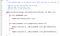classify method

# Video

https://vimeo.com/535684281

# Conclusion

Thank you for taking the time to read through my blog post and I hope you have learned something new about machine learning. Now that we have covered the main parts of the decision tree classifier, I invite you to test it out for yourself and try and modify it and make it better than I did. I have uploaded all of the code to github so you can play with it and learn from it. I have also linked some useful online resources below that helped me learn and understand some Machine Learning concepts.

# References

https://www.guru99.com/supervised-vs-unsupervised-learning.html

https://www.analyticsvidhya.com/blog/2017/09/common-machine-learning-algorithms/

https://www.youtube.com/playlist?list=PLOU2XLYxmsIIuiBfYad6rFYQU_jL2ryal

https://machinelearningmastery.com/types-of-learning-in-machine-learning/

https://towardsdatascience.com/gini-impurity-measure-dbd3878ead33

https://victorzhou.com/blog/information-gain/